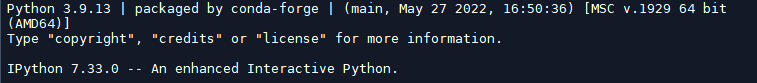##### replace sets of values in an array without using loops
 replace sets of values in an array without using loops paul18fr Spam, spam, eggs, and spam Posts: 206 Threads: 53 Joined: Apr 2019 Reputation: Jun-19-2022, 09:28 PM Hi, I've been thinking I've ever done this, but I do not remember how; in any way I do not understand the Type Error sine I'm using intergers: what I'm missing? Thanks ```import numpy as np A=np.array([1, 2, 3]) M=np.zeros((10,10), dtype=float) N=np.copy(M) P = np.copy(M) # Explicitly on 1 location r=3 c=2 M[r, c:c+3]=A # now on 2 locations # with a loop r=np.array([3, 5]) c=np.array([2, 5]) for i in range(2): N[r[i], c[i]:c[i]+3]=A #without a loop i=np.arange(2) j=c[i]+3*np.ones(2, dtype=int) k = np.ones((2,1), dtype=int)*A P[r[i], c[i]:j[i]]=k[i]`````Error:TypeError: only integer scalar arrays can be converted to a scalar index`` Reply Larz60+ aetate et sapientiaPosts: 11,561 Threads: 446 Joined: Sep 2016 Reputation: Jun-19-2022, 10:02 PM you won't get a type error unless their really is one. Please show complete, unaltered error traceback (always), it includes valuable program execution order information. Reply paul18fr Spam, spam, eggs, and spam Posts: 206 Threads: 53 Joined: Apr 2019 Reputation: Jun-20-2022, 06:16 AM Hi Larz60+ As requested: ``````Error:runfile('C:/forum.py', wdir='C:/MyDir') Traceback (most recent call last): File "C:\outils\Anaconda3\lib\site-packages\spyder_kernels\py3compat.py", line 356, in compat_exec exec(code, globals, locals) File "c:/forum.py", line 26, in P[r[i], c[i]:j[i]]=k[i] TypeError: only integer scalar arrays can be converted to a scalar index`````` Reply Larz60+ aetate et sapientiaPosts: 11,561 Threads: 446 Joined: Sep 2016 Reputation: Jun-20-2022, 08:29 AM You can write the code as follows to display values if TypeError encountered: ```import numpy as np import sys A = np.array([1, 2, 3]) M = np.zeros((10, 10), dtype=float) N = np.copy(M) P = np.copy(M) # Explicitly on 1 location r = 3 c = 2 M[r, c : c + 3] = A print(f"\nM(r, c:c_3): {M[r, c:c+3]}") # now on 2 locations # with a loop r = np.array([3, 5]) c = np.array([2, 5]) for i in range(2): try: N[r[i], c[i] : c[i] + 3] = A except TypeError: print(f"First loop: Values when TypeError occurs:, i: {i}, c[i]: {c[i]}") sys.exit(-1) # without a loop i = np.arange(2) j = c[i] + 3 * np.ones(2, dtype=int) k = np.ones((2, 1), dtype=int) * A try: P[r[i], c[i] : j[i]] = k[i] except TypeError: print( f"\nTypeError, Values when attempting P[r[i], c[i]:j[i]]=k[i]:\n" f" i: {i}, r[i]: {r[i]}, c[i]: {c[i]}, j[i]: {j[i]}" ) sys.exit(-1)```output shows where error occurs: ``````Output:M(r, c:c_3): [1. 2. 3.] TypeError, Values when attempting P[r[i], c[i]:j[i]]=k[i]: i: [0 1], r[i]: [3 5], c[i]: [2 5], j[i]: [5 8]`````` Reply paul18fr Spam, spam, eggs, and spam Posts: 206 Threads: 53 Joined: Apr 2019 Reputation: Jun-20-2022, 08:47 AM Ok I keep it in mind (I know that I need to implement more Exceptions in my code). Nonetheless I'm here looking for a way to not use a loop to slice following columns; in the example hereafter, it works with a single arugument/tuple, but I cannot go further Paul ```#without a loop r=np.array([3, 5]) c=np.array([2, 5]) i=np.arange(2) d=c[i]+3*np.ones(2, dtype=int) k = np.ones((2,1), dtype=int)*A # P[r[i], 2:5]=k[i] # ok P[r[i], slice(2, 5)]=k[i] # ok``` Reply paul18fr Spam, spam, eggs, and spam Posts: 206 Threads: 53 Joined: Apr 2019 Reputation: Jun-20-2022, 11:59 AM After some tests and trials, I can say that the issue comes from the Slice object, but I still trying to understand how to fix it. ```import numpy as np import sys A=np.array([1, 2, 3]) M=np.zeros((10,10), dtype=float) N=np.copy(M) P=np.copy(M) # # Explicitly on 1 location r=3 c=2 M[r, c:c+3]=A # now on 2 locations # with a loop r=np.array([3, 5]) c=np.array([2, 5]) for i in range(2): N[r[i], c[i]:c[i]+3]=A #without a loop i=np.arange(2) d=c[i]+3*np.ones(2, dtype=int) SliceIndexes = np.vstack((c,d)) k = np.ones((2,1), dtype=int)*A SliceObject = slice(SliceIndexes[i], SliceIndexes[i]) P[r[i], SliceObject ]=k[i] # ko # P[r[i], 0:3]=k[i] # ok # P[r[i], slice(2,5)]=k[i] # ok```If somebody knows how to, it'll be highly appreciated. Paul Here bellow the screenshot of information gotten under Spyder:Reply Larz60+ aetate et sapientiaPosts: 11,561 Threads: 446 Joined: Sep 2016 Reputation: Jun-20-2022, 07:49 PM Which version of python are you running? from command line: `python -V` Reply paul18fr Spam, spam, eggs, and spam Posts: 206 Threads: 53 Joined: Apr 2019 Reputation: Jun-20-2022, 08:15 PM Hi Larz60+ Python 3.9.13Reply

 Possibly Related Threads… Thread Author Replies Views Last Post Data sets comparison Fraetos 0 940 Sep-14-2021, 06:45 AM Last Post: Fraetos Keep inner Values of 2D array timste 0 1,065 Jul-26-2021, 09:04 AM Last Post: timste Mann Whitney U-test on several data sets rybina 2 1,471 Jan-05-2021, 03:08 PM Last Post: rybina Least-squares fit multiple data sets multiverse22 1 1,827 Jun-06-2020, 01:38 AM Last Post: Larz60+ replace nan values by mean group by date.year, date.month wissam1974 5 6,638 Feb-19-2020, 06:25 PM Last Post: AnkitGupta Identifying consecutive masked values in a 3D data array chai0404 12 4,272 Feb-01-2020, 12:59 PM Last Post: perfringo Clustering for imbalanced data sets dervast 0 1,195 Sep-25-2019, 06:34 AM Last Post: dervast Trying to replace for loops with numpy vspoloni 8 7,881 Aug-04-2019, 07:09 AM Last Post: paul18fr Match two data sets based on item values klllmmm 7 5,247 Mar-29-2017, 02:33 PM Last Post: zivoni

Forum Jump:

### User Panel Messages##### Announcements
Announcement #1 8/1/2020
Announcement #2 8/2/2020
Announcement #3 8/6/2020